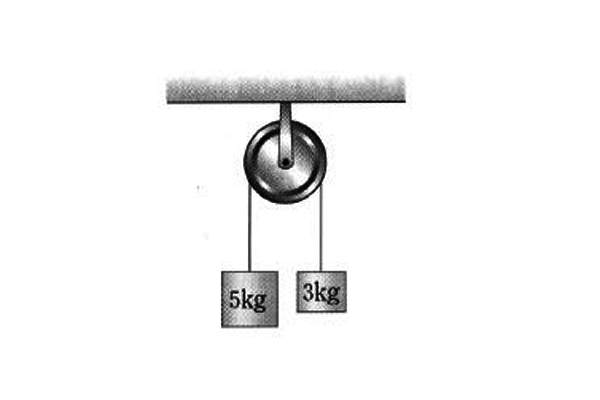# Acceleration with pulleyIn the above diagram, two objects with their respective masses $5$ kg and $3$ kg are hung on a pulley with no friction. Find the magnitude of acceleration $(\text{in m/s}^2 )$ of the left object with mass $5$ kg.

Gravitational acceleration is $g = 10$ m/s$^{2}$.

×